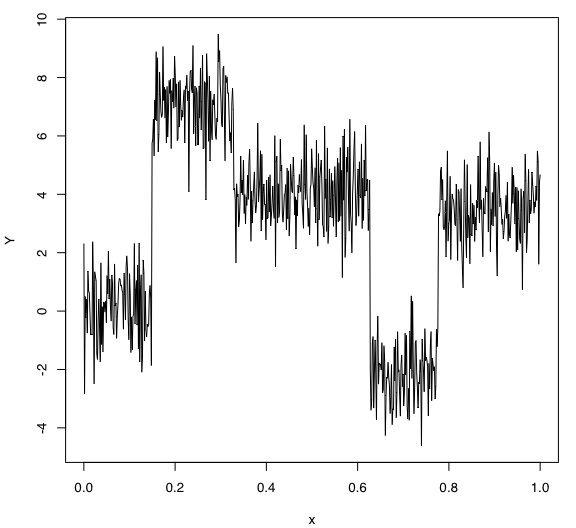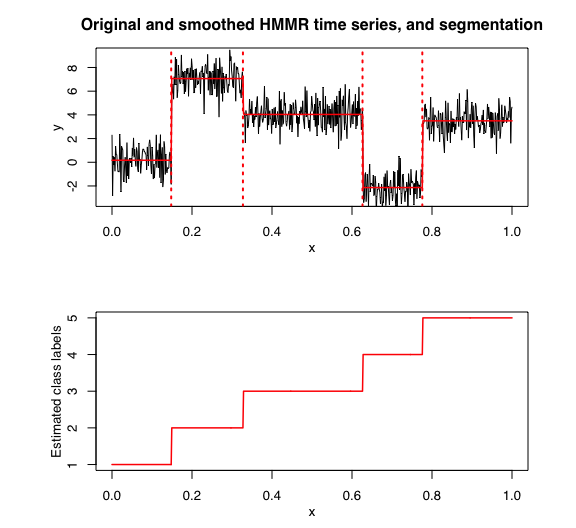# Introduction

In this package, it is possible to select models based on information criteria such as BIC, AIC and ICL.

The selection is done on two parameters which are:

• $$K$$: The number of regimes;
• $$p$$: The order of the polyniomial regression.

# Data

Let’s select a RHLP model for the following time series $$Y$$:

data("univtoydataset")
x <- univtoydataset$x y <- univtoydataset$y
plot(x, y, type = "l", xlab = "x", ylab = "Y")# Model selection with BIC

selectedhmmr <- selectHMMR(X = x, Y = y, Kmin = 2, Kmax = 6, pmin = 0, pmax = 3)
## The HMMR model selected via the "BIC" has K = 5 regimes
##  and the order of the polynomial regression is p = 0.
## BIC = -1136.39152222095
## AIC = -1059.76780111041

The selected model has $$K = 5$$ regimes and the order of the polynomial regression is $$p = 0$$. According to the way $$Y$$ has been generated, these parameters are what we expected.

Let’s summarize the selected model:

selectedhmmr$summary() ## --------------------- ## Fitted HMMR model ## --------------------- ## ## HMMR model with K = 5 components: ## ## log-likelihood nu AIC BIC ## -1025.768 34 -1059.768 -1136.392 ## ## Clustering table (Number of observations in each regimes): ## ## 1 2 3 4 5 ## 100 120 200 100 150 ## ## Regression coefficients: ## ## Beta(K = 1) Beta(K = 2) Beta(K = 3) Beta(K = 4) Beta(K = 5) ## 1 0.1694566 7.063444 4.036769 -2.134901 3.49582 ## ## Variances: ## ## Sigma2(K = 1) Sigma2(K = 2) Sigma2(K = 3) Sigma2(K = 4) Sigma2(K = 5) ## 1.268478 1.126648 1.086297 1.011927 1.046276 selectedhmmr$plot(what = "smoothed")